NEET  >  NEET Previous Year Questions (2014-21): Structure of Atom

# NEET Previous Year Questions (2014-21): Structure of Atom Notes | Study Chemistry Class 11 - NEET

## Document Description: NEET Previous Year Questions (2014-21): Structure of Atom for NEET 2022 is part of Structure of Atom for Chemistry Class 11 preparation. The notes and questions for NEET Previous Year Questions (2014-21): Structure of Atom have been prepared according to the NEET exam syllabus. Information about NEET Previous Year Questions (2014-21): Structure of Atom covers topics like and NEET Previous Year Questions (2014-21): Structure of Atom Example, for NEET 2022 Exam. Find important definitions, questions, notes, meanings, examples, exercises and tests below for NEET Previous Year Questions (2014-21): Structure of Atom.

Introduction of NEET Previous Year Questions (2014-21): Structure of Atom in English is available as part of our Chemistry Class 11 for NEET & NEET Previous Year Questions (2014-21): Structure of Atom in Hindi for Chemistry Class 11 course. Download more important topics related with Structure of Atom, notes, lectures and mock test series for NEET Exam by signing up for free. NEET: NEET Previous Year Questions (2014-21): Structure of Atom Notes | Study Chemistry Class 11 - NEET
 1 Crore+ students have signed up on EduRev. Have you?

Q.1. A particular station of All India Radio, New Delhi, broadcasts on a frequency of 1,368 kHz (kilohertz). The wavelength of the electromagnetic radiation emitted by the transmitter is : [speed of light, c = 3.0 x 108 ms-1]     (2021)
(a) 2192 m
(b) 21.92 cm
(c) 219.3 m
(d) 219.2 m
Ans.
c

The Vividh Bharati station of All India Radio, Delhi, broadcasts on a frequency of 1,368 kHz (kilo hertz).

Q.2. The number of protons, neutrons and electrons in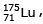respectively, are :     (2020)
(a) 71, 71 and 104
(b) 175, 104 and 71
(c) 71, 104 and 71
(d) 104, 71 and 71

Ans: c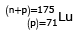∴ no. of protons = 71
no. of neutrons = 175 – 71 = 104
no. of electrons = 71

Q.3. 4d, 5p, 5f and 6p orbitals are arranged in the order of decreasing energy. The correct option is    (2019)
(a) 5f > 6p > 5p > 4d

(b) 6p > 5f > 5p > 4d
(c) 6p > 5f > 4d > 5p
(d) 5f > 6p > 4d > 5p
Ans:
a

(n + l) values for,
4d = 4 + 2 = 6
5p = 5 + 1 = 6
5f = 5 + 3 = 8
6p = 6 + 1 = 7
∴ Correct order of energy would be
5f > 6p > 5p > 4d

Q.4. Which of the following series of transitions in the spectrum of hydrogen atom fall in visible region?    (2019)
(a) Lyman series
(b) Balmer series
(c) Paschen series
(d) Brackett series
Ans:
b

In H-spectrum, Balmer series transitions fall in visible region.

Q.5. Which one is a wrong statement ?    (2018)
(a) Total orbital angular momentum of electron in 's' orbital is equal to zero
(b) An orbital is designated by three quantum numbers while an electron in an atom is designated by four quantum numbers.
(c) The electronic configuration of N atom is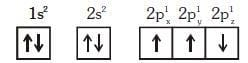(d) The value of m for dz2 is zero
Ans:
c

The correct configuration of 'N' is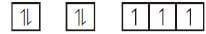Q.6. Match the metal ions given in Column I with the spin magnetic moments of the ions given in Column II and assign the correct code :    (2018)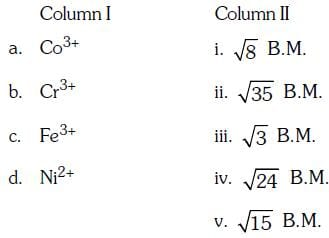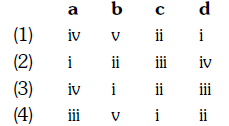(a) 1
(b) 2
(c) 3
(d) 4
Ans:
a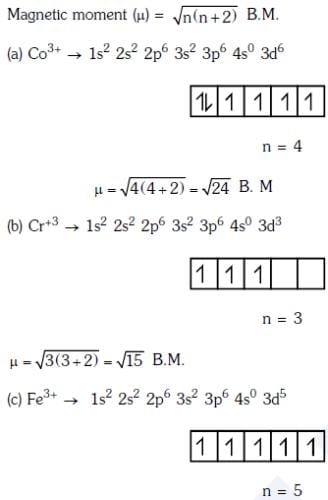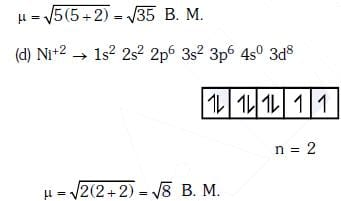Q.7. Two electrons occupying the same orbital are distinguished by :    (2016)
(a) Spin quantum number
(b) Principal quantum number
(c) Magnetic quantum number
(d) Azimuthal quantum number
Ans:
a

Same orbital can have two different values of spin of e- of +1/2 and -1/2 (spin quantum number)

Q.8. The angular momentum of electron in 'd' orbital is equal to:    (2015)
(a) 0 h
(b) √6h
(c) √2h
(d) 2√3h
Ans:
b

Angular momentum of electron in 'd‘ orbital =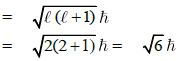Q.9. Magnetic moment 2.84 B.M. is given by : (At. Nos. Ni = 28, Ti = 22, Cr = 24, Co = 27)    (2015)
(a) Co2+
(b) Ni2+
(c) Ti3+
(d) Cr2+
Ans:
b

Magnetic moment =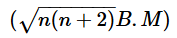= 2.84 i.e., n = 2
Ni+2 i.e., 3d8 contains two unpaired electrons

Q.10. The number of d-electrons in Fe2+ (Z = 26) is not equal to the number of electrons in which one of the following ?    (2015)
(a) p - electrons in Ne (Z = 10)
(b) s - electrons in Mg (Z = 12)
(c) p - electrons in Cl (Z = 17)
(d) d - electrons in Fe (Z = 26)
Ans:
c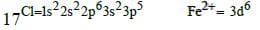Q.11. Magnetic moment 2.83 BM is given by which of the following ions ?
(At. Nos. Ti = 22, Cr = 24, Mn = 25, Ni = 28)    (2014)
(a) Cr3+
(b) Mn2+
(c) Ti3+
(d) Ni2+
Ans:
d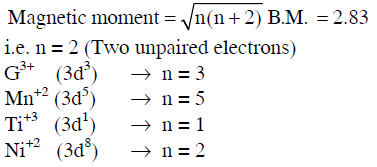Q.12. What is the maximum number of orbitals that can be identified with the following quantum numbers ? n = 3,l= 1, ml = 0    (2014)
(a) 3
(b) 4
(c) 1
(d) 2
Ans:
c

3p orbital can have n = 3, = 1 and ml = 0.

Q.13. Calculate the energy in joule corresponding to light of wavelength 45 nm :
(Planck's constant h = 6.63 × 10−34 Js; speed of light c = 3 × 108 ms−1)    (2014)
(a) 4.42 × 10-15
(b) 4.42 × 10-18
(c) 6.67 × 1015

(d) 6.67 × 1011

Ans: b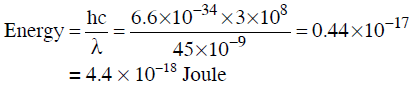The document NEET Previous Year Questions (2014-21): Structure of Atom Notes | Study Chemistry Class 11 - NEET is a part of the NEET Course Chemistry Class 11.
All you need of NEET at this link: NEET

## Chemistry Class 11

204 videos|320 docs|229 tests
 Use Code STAYHOME200 and get INR 200 additional OFF

## Chemistry Class 11

204 videos|320 docs|229 tests

### How to Prepare for NEET

Read our guide to prepare for NEET which is created by Toppers & the best Teachers

Track your progress, build streaks, highlight & save important lessons and more!

,

,

,

,

,

,

,

,

,

,

,

,

,

,

,

,

,

,

,

,

,

;